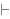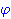Intuitionistic Logic Explorer < Previous   Next > Nearby theorems Mirrors  >  Home  >  ILE Home  >  Th. List  >  conventions Unicode version

Theorem conventions 10819
Description: Unless there is a reason to diverge, we follow the conventions of the Metamath Proof Explorer (aka "set.mm"). This list of conventions is intended to be read in conjunction with the corresponding conventions in the Metamath Proof Explorer, and only the differences are described below.
• Minimizing axioms and the axiom of choice. We prefer proofs that depend on fewer and/or weaker axioms, even if the proofs are longer. In particular, our choice of IZF (Intuitionistic Zermelo-Fraenkel) over CZF (Constructive Zermelo-Fraenkel, a weaker system) was just an expedient choice because IZF is easier to formalize in Metamath. You can find some development using CZF in BJ's mathbox starting at wbd 10870 (and the section header just above it). As for the axiom of choice, the full axiom of choice implies excluded middle as seen at acexmid 5562, although some authors will use countable choice or dependent choice. For example, countable choice or excluded middle is needed to show that the Cauchy reals coincide with the Dedekind reals - Corollary 11.4.3 of [HoTT], p. (varies).
• Junk/undefined results. Much of the discussion of this topic in the Metamath Proof Explorer applies except that certain techniques are not available to us. For example, the Metamath Proof Explorer will often say "if a function is evaluated within its domain, a certain result follows; if the function is evaluated outside its domain, the same result follows. Since the function must be evaluated within its domain or outside it, the result follows unconditionally" (the use of excluded middle in this argument is perhaps obvious when stated this way). For this reason, we generally need to prove we are evaluating functions within their domains and avoid the reverse closure theorems of the Metamath Proof Explorer.
• Bibliography references. The bibliography for the Intuitionistic Logic Explorer is separate from the one for the Metamath Proof Explorer but feel free to copy-paste a citation in either direction in order to cite it.

Label naming conventions

Here are a few of the label naming conventions:

• Suffixes. We follow the conventions of the Metamath Proof Explorer with a few additions. A biconditional in set.mm which is an implication in iset.mm should have a "r" (for the reverse direction), or "i"/"im" (for the forward direction) appended. A theorem in set.mm which has a decidability condition added should add "dc" to the theorem name. A theorem in set.mm where "nonempty class" is changed to "inhabited class" should add "m" (for member) to the theorem name.

The following table shows some commonly-used abbreviations in labels which are not found in the Metamath Proof Explorer, in alphabetical order. For each abbreviation we provide a mnenomic to help you remember it, the source theorem/assumption defining it, an expression showing what it looks like, whether or not it is a "syntax fragment" (an abbreviation that indicates a particular kind of syntax), and hyperlinks to label examples that use the abbreviation. The abbreviation is bolded if there is a df-NAME definition but the label fragment is not NAME.

AbbreviationMnenomicSource ExpressionSyntax?Example(s)
apapart df-ap 7801 Yes apadd1 7827, apne 7842

• Community. The Metamath mailing list also covers the Intuitionistic Logic Explorer and is at: https://groups.google.com/forum/#!forum/metamath.
• (Contributed by Jim Kingdon, 24-Feb-2020.)

Hypothesis
Ref Expression
conventions.1Assertion
Ref Expression
conventionsProof of Theorem conventions
StepHypRef Expression
1 conventions.1 1Colors of variables: wff set class This theorem is referenced by: (None)
 Copyright terms: Public domain W3C validator